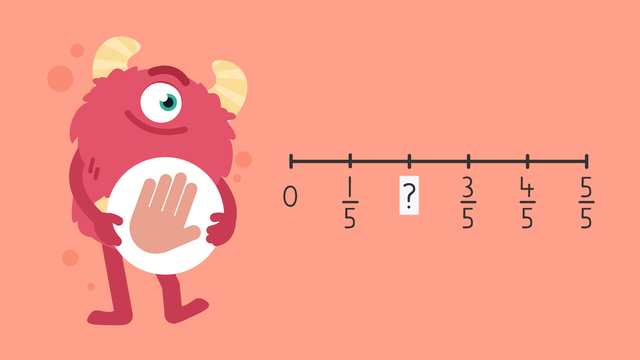# Identify Missing Fractions on a Number Line — Let's Practice!Rating

Ø 4.7 / 3 ratings
The authorsTeam Digital

## Basics on the topicIdentify Missing Fractions on a Number Line — Let's Practice!

Today we are practicing identifying missing fractions on a number line with Razzi! This video contains examples to help you further practice and grow confident in this topic.

### TranscriptIdentify Missing Fractions on a Number Line — Let's Practice!

Razzi says get these items ready (...) Because today we're going to... Identify Missing Fractions on a Number Line. It's time to begin! Identify the missing fraction on the number line. Pause the video to work on the problem (...) and press play when you are ready to see the solution! First, find the interval by identifying the pattern between the given fractions. Then, count-on using the pattern starting with the first fraction. Did you also get two-fifths? Let's keep practicing! Identify the missing fraction on the number line. Pause the video to work on the problem (...) and press play when you are ready to see the solution! First, find the interval by identifying the pattern between the given fractions. Then, count backwards from seven-twelfths. Did you also get four-twelfths? Let's do one more! This time, identify ALL the missing fractions on the number line. Pause the video to work on the problem (...) and press play when you are ready to see the solution! There are seven parts of one whole, so the denominator is seven... then starting with one-seventh, fill in the missing numerators. Did you also get one-seventh, two-sevenths, three-sevenths, four-sevenths, five-sevenths, six-sevenths, and seven-sevenths? Razzi had so much fun practicing with you today! See you next time

## Identify Missing Fractions on a Number Line — Let's Practice! exercise

Would you like to apply the knowledge you’ve learned? You can review and practice it with the tasks for the video Identify Missing Fractions on a Number Line — Let's Practice!.
• ### Razi's missing fractions.

Hints

Find the interval by identifying the pattern between the given fractions.

Count on using the pattern starting with the first fraction.

Solution

The missing fraction on the number line is $\frac{3}{6}$.

• ### More missing fractions.

Hints

How many parts of one whole are on the number line? That is your denominator.

$\frac{1}{9}$ belongs after 0. $\frac{2}{9}$ is next. Use this pattern to determine the missing fraction.

Solution

$\frac{4}{9}$ is the missing fraction.

• ### The number line is missing fractions!

Hints

How many parts of one whole are on the number line? That is your denominator.

Continue counting along the number line to find the missing fractions.

Solution

The image shows the correct fractions on the number line.

• ### Find the missing fractions.

Hints

Use the pattern to find the missing fractions.

Count on or back from $\frac{9}{14}$ to find the missing fractions.

Solution

The missing fractions are $\frac{1}{14}$ and $\frac{5}{14}$.

• ### Fractions.

Hints

Which fraction comes after $\frac{2}{4}$?

Which fraction comes before $\frac{4}{4}$?

Solution

The missing fraction is $\frac{3}{4}$.

• ### Measure flour to help Razzi bake cookies.

Hints

What is the denominator?

Begin at $\frac{1}{5}$ on the number line. Add $\frac{3}{5}$ more.

Solution
• Begin at $\frac{1}{5}$ and make 3 more hops to add $\frac{3}{5}$
• You land at $\frac{4}{5}$
• Razzi needs $\frac{4}{5}$ cup of flour.